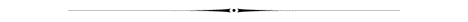More will be added as time permits.WEIGHTS:(Aviordupois)
1 milligram=0.001 gram
1 gram= 1000 milligrams=
1 kilogram=1000 grams =35.27 ounces
1 ounce= 28.3 grams= 16 drams= 437.5 grains=28.350 grams
1 pound= 16 ounces= 7000 grains= 454 grams
1 ton(short)= 2000 pounds= 20 shortweight= 0.907 metric ton= 907.2 kilograms
1 ton (long)= 2240 pounds= 20 longweight = 1.016 metric tons
1 short hundredweight= 100 pounds= 0.5 short ton
1 long hundredweight= 112 pounds= 0.05 long ton

Weights:(Troy)
1 pound= 12 ounces= 240 pennyweight= 5760 grains
1 ounce= 20 pennyweight= 480 grains= 0.083 pound
1 pennyweight= 24 grains= 0.05 ounce
1 grain= 0.042 pennyweight= 0.05 scrple= 0.002083 ounce= 0.0166 dram

Weights:(Apothecaries)
1 pound= 12 ounces= 5760 grains
1 ounce= 8 drams= 480 grains= 0.083 pounds= 31.103 grams
1 dram= 3 scruples= 60 grains
1 scruple= 20 grains= 0.333 drams
1 grain= 0.05 scruples= 0.002083 ounces= 0.0166 dram

Area:
1 square inch= 0.0069 square foot= 0.00077 square yard
1 square foot=144 square inches= 0.111 square yard
1 square yard=9 square feet=1296 square inches
1 square rod= 30.25 square yards= 0.00625 acre
1 square acre=43560 square feet=4840 square yards
1 square mile=640 acres
1 township=36 square miles
1 hectare=2.472 acres=107635 square feet
1 square meter=10.746 square feet

MEASUREMENTS
 1 inch (in.) = 25.40 millimeters (mm) 1 sq. inch (in.2) = 645.16 square millimeters 1 sq. foot (ft.2) = 0.092903 square meter 1 millimeter (mm) = 0.03937 inch 1 sq. millimeter (mm2) = 0.00155 sq. inch 1 meter (m) = 3.2808 feet OR 1.09361 yards 1 sq. meter (m2) = 10.7639 sq. feet OR 1.196 sq. yards

Distance
1 inch= 0.083 feet= 0.28 yards= 2.54 centimeters
1 foot= 12 inches= 0.333 yard
1 yard= 3 feet= 36 inches= 0.9144 meter
1 rod= 16.5 feet= 5.50 yard= 5.029 meters
1 mile= 1760 yards=5280 feet=320 rods= 63360 inches
1 mile= 1.609 kilometers= 1609.3 meters= 160930 centimeters
1 kilometer= 0.6214 miles= 3281 feet
1 kilometer= 1093.6 yards

Volume:
1 gallon= 3785 cubic centimeters
1 gallon= 0.1337 cubic feet=231 cubic inches
1 bushel=1.244 cubic feet= 2150 cubic inches
1 bushel= 64 pints=32 quarts
1 bushel= 0.04545 cubic yards
1 cubic inch= 0.00058 cubic foot= 0.000021 cubic yard
1 cubic foot= 1728 cubic inches= 0.0370 cubic yard
1 cubic yard= 27 cubic feet
1 cubic yard= 46656 cubic inches
1 cubic yard= 764.6 liters= 202 gallons
1 cubic yard= 807.9 quarts= 1616 pints
1 cubic yard= 764600 cubic centimeters

Liquid volume
1 level tablespoon=3 level teaspoon
1 fluid ounce= 2 tablespoons=29.573 milliliters
1 cup= 8 fluid ounces
1 pint= 2 cups=16 fluid ounces
1 pint= 4 gills= 28.875 cubic inches
1 gill= 4 fluid ounces= 7.219 cubic inches
1 quart= 2 pints=32 fluid ounces
1 gallon= 4 quarts= 128 fluid ounces
1 gallon= 231 cubic inches